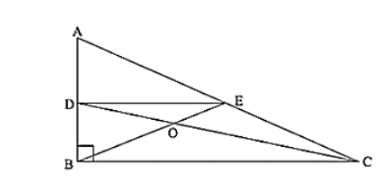Deepak Scored 45->99%ile with Bounce Back Crack Course. You can do it too!

# In figure, Triangle ABC is a right angled triangle at B.

Question:

In figure, Triangle ABC is a right angled triangle at B. Given that AB = 9 cm, AC = 15 cm and D, E are the mid-points of the sides AB and AC respectively, calculate

(i) The length of BC

Solution:

In ΔABC, ∠B = 900,

By using Pythagoras theorem

$A C^{2}=A B^{2}+B C^{2}$

$\Rightarrow 15^{2}=9^{2}+B C^{2}$

$\Rightarrow B C=\sqrt{15^{2}-9^{2}}$

$\Rightarrow \mathrm{BC}=\sqrt{225-81}$

$\Rightarrow \mathrm{BC}=\sqrt{144}=12 \mathrm{~cm}$

In ΔABC,

D and E are mid-points of AB and AC

∴ DE ∥ BC, DE = (1/2) BC [By mid−point theorem]

AD = DB = AB/2 = 9/2 = 4.5 cm [∵ D is the mid−point of AB]]

$=13.5 \mathrm{~cm}^{2}$